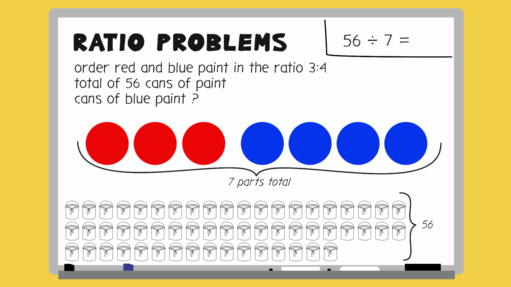Science, Maths & Technology

### Become an OU studentEveryday maths 2

Start this free course now. Just create an account and sign in. Enrol and complete the course for a free statement of participation or digital badge if available.

# 7.1 Solving ratio problems where the total is given

The best way for you to understand how to solve these problems is to look through the worked example in the video below.Interactive feature not available in single page view (see it in standard view).

## Activity 13: Ratio problems where the total is given

Try solving these ratio problems:

1. To make mortar you need to mix soft sand and cement in the ratio 4:1. You need to make a total of 1500 g of mortar. How much soft sand will you need?
2. To make the mocktail ‘Sea Breeze’, you need to mix cranberry juice and grapefruit juice in the ratio 4:2. You want to make a total of 2700 ml of mocktail. How much grapefruit juice should you use?

1. Add the parts of the ratio:
• 4 + 1 = 5

Divide the total amount required by the sum of the parts of the ratio:

• 1500 g ÷ 5 = 300 g

Since soft sand is 4 parts, we do 300 g × 4 = 1200 g of soft sand.

2. Add the parts of the ratio:

• 4 + 2 = 6

Divide the total amount required by the sum of the parts of the ratio:

• 2700 ml ÷ 6 = 450 ml

Since grapefruit juice is 2 parts, we do 450 ml × 2 = 900 ml of grapefruit juice.